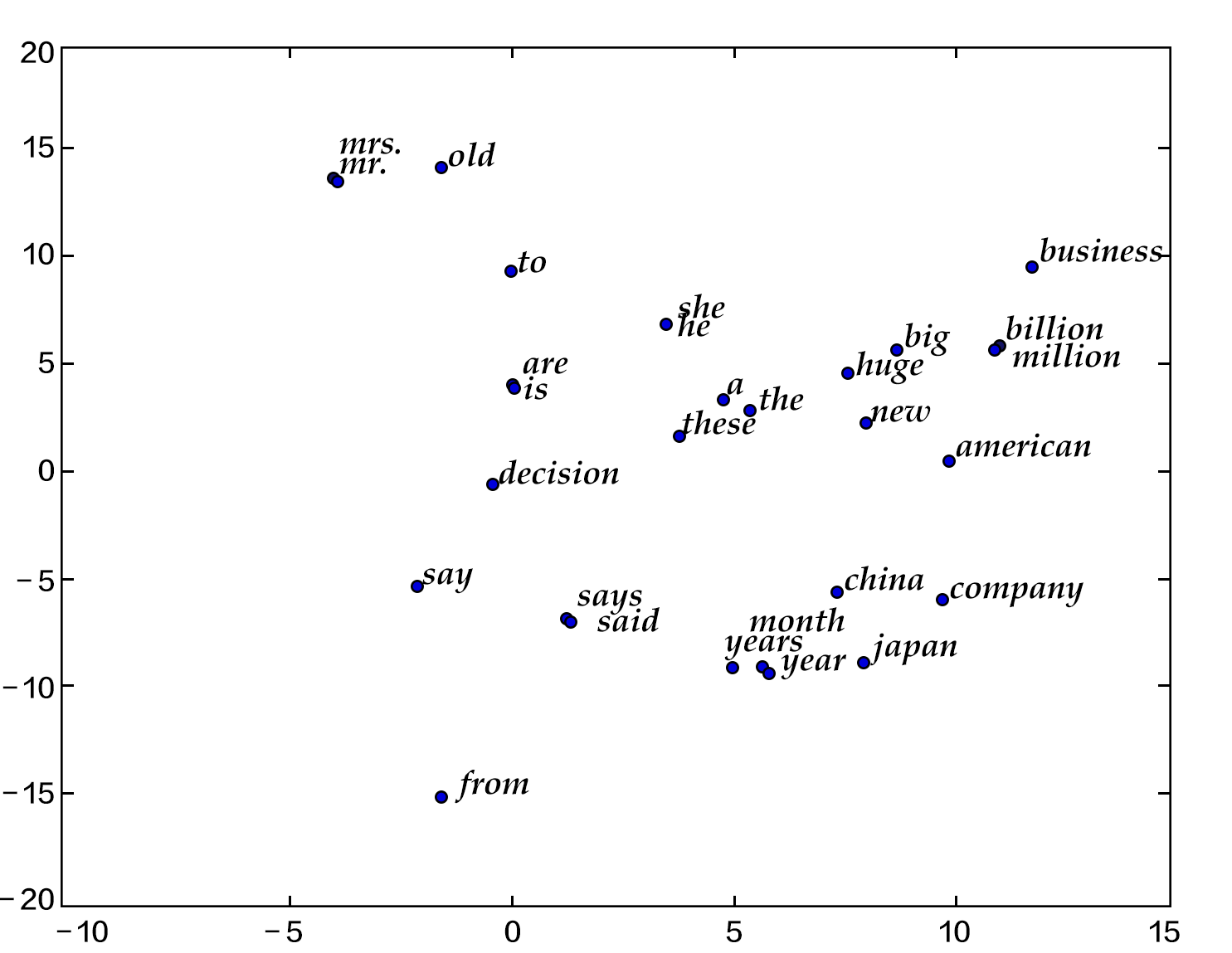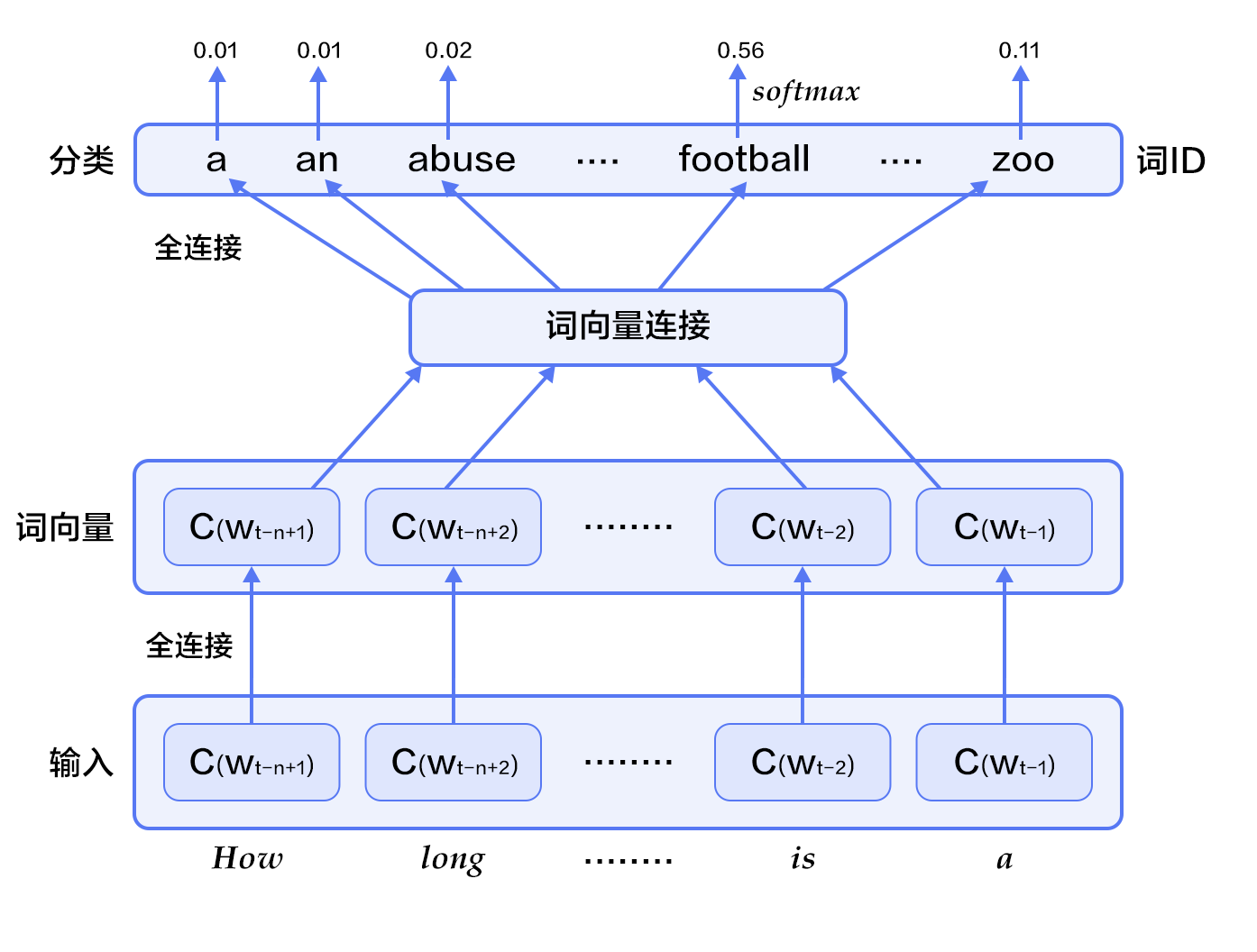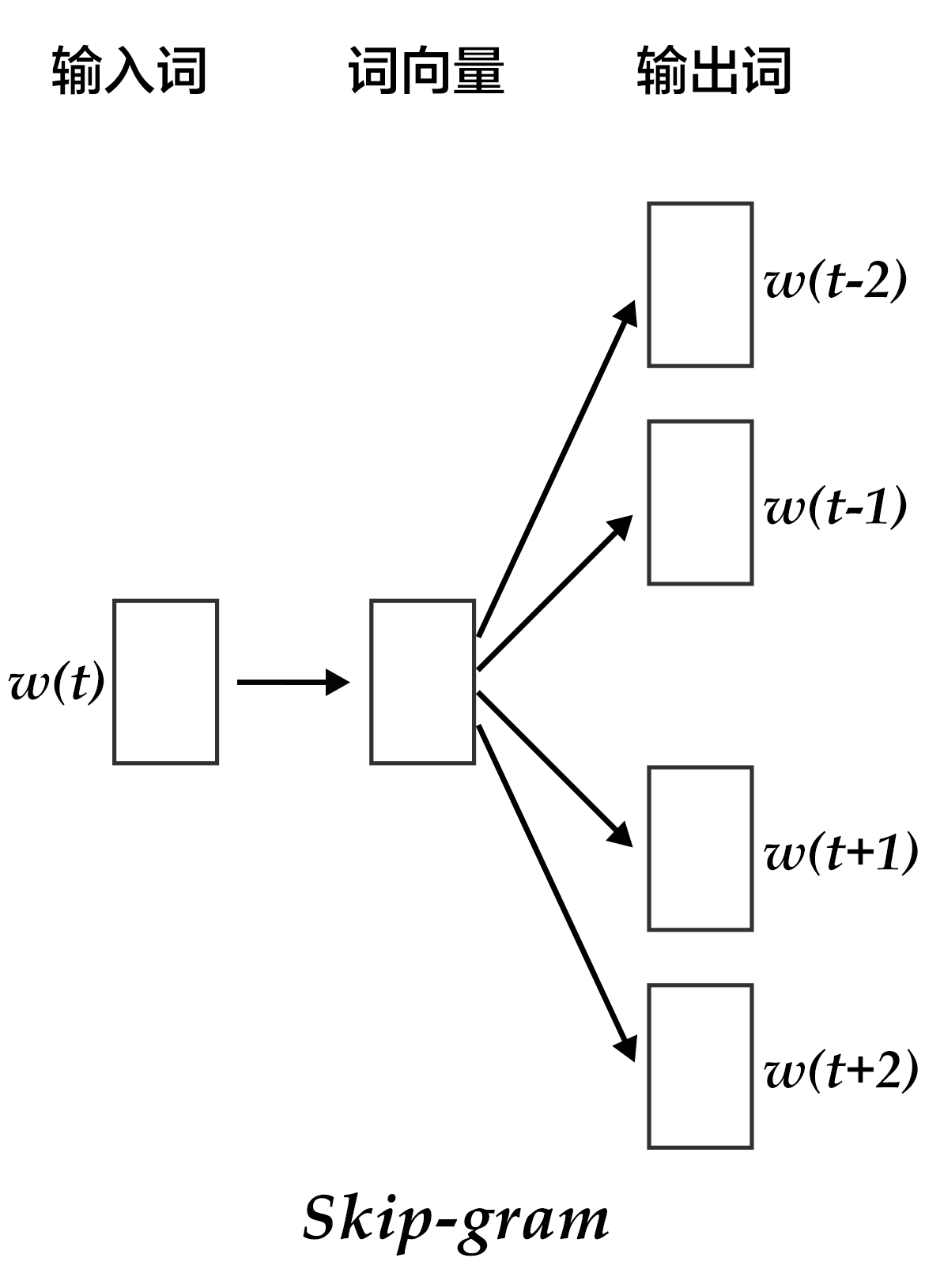# 词向量

## 背景介绍

One-hot vector虽然自然，但是用处有限。比如，在互联网广告系统里，如果用户输入的query是“母亲节”，而有一个广告的关键词是“康乃馨”。虽然按照常理，我们知道这两个词之间是有联系的——母亲节通常应该送给母亲一束康乃馨；但是这两个词对应的one-hot vectors之间的距离度量，无论是欧氏距离还是余弦相似度(cosine similarity)，由于其向量正交，都认为这两个词毫无相关性。 得出这种与我们相悖的结论的根本原因是：每个词本身的信息量都太小。所以，仅仅给定两个词，不足以让我们准确判别它们是否相关。要想精确计算相关性，我们还需要更多的信息——从大量数据里通过机器学习方法归纳出来的知识。

X=USVT

1) 由于很多词没有出现，导致矩阵极其稀疏，因此需要对词频做额外处理来达到好的矩阵分解效果；

2) 矩阵非常大，维度太高(通常达到 106106 $10^6*10^6$的数量级)；

3) 需要手动去掉停用词（如although, a,…），不然这些频繁出现的词也会影响矩阵分解的效果。

## 效果展示similarity: 0.899180685161
please input two words: big huge

please input two words: from company
similarity: -0.0997506977351

## 模型概览

### 语言模型

P(w1,...,wT)=t=1TP(wt)

P(w1,...,wT)=t=1TP(wt|w1,...,wt1)
### N-gram neural model 在计算语言学中，n-gram是一种重要的文本表示方法，表示一个文本中连续的n个项。基于具体的应用场景，每一项可以是一个字母、单词或者音节。 n-gram模型也是统计语言模型中的一种重要方法，用n-gram训练语言模型时，一般用每个n-gram的历史n-1个词语组成的内容来预测第n个词。 Yoshua Bengio等科学家就于2003年在著名论文 Neural Probabilistic Language Models
(#参考文献)

P(w1,...,wT)=t=nTP(wt|wt1,wt2,...,wtn+1)

1Ttf(wt,wt1,...,wtn+1;θ)+R(θ)- 对于每个样本，模型输入 wtn+1,...wt1 $w_{t-n+1},...w_{t-1}$, 输出句子第t个词为字典中|V|个词的概率。

• 然后所有词语的词向量连接成一个大向量，并经过一个非线性映射得到历史词语的隐层表示：

g=Utanh(θTx+b1)+Wx+b2

其中， x $x$为所有词语的词向量连接成的大向量，表示文本历史特征；θ$\theta$ U $U$b1$b_1$ b2 $b_2$ W $W$分别为词向量层到隐层连接的参数。g$g$表示未经归一化的所有输出单词概率， gi $g_i$表示未经归一化的字典中第 i $i$个单词的输出概率。

• 根据softmax的定义，通过归一化gi$g_i$, 生成目标词 wt $w_t$的概率为：

P(wt|w1,...,wtn+1)=egwt|V|iegi

• 整个网络的损失值(cost)为多类分类交叉熵，用公式表示为

J(θ)=i=1Nc=1|V|yiklog(softmax(gik))

其中 yik $y_k^i$表示第 i $i$个样本第k$k$类的真实标签(0或1)， softmax(gik) $softmax(g_k^i)$表示第i个样本第k类softmax输出的概率。

### Continuous Bag-of-Words model(CBOW)

CBOW模型通过一个词的上下文（各N个词）预测当前词。当N=2时，模型如下图所示：context=xt1+xt2+xt+1+xt+24

### Skip-gram model

CBOW的好处是对上下文词语的分布在词向量上进行了平滑，去掉了噪声，因此在小数据集上很有效。而Skip-gram的方法中，用一个词预测其上下文，得到了当前词上下文的很多样本，因此可用于更大的数据集。## 参考文献

1. Bengio Y, Ducharme R, Vincent P, et al. A neural probabilistic language model[J]. journal of machine learning research, 2003, 3(Feb): 1137-1155.
2. Mikolov T, Kombrink S, Deoras A, et al. Rnnlm-recurrent neural network language modeling toolkit[C]//Proc. of the 2011 ASRU Workshop. 2011: 196-201.
3. Mikolov T, Chen K, Corrado G, et al. Efficient estimation of word representations in vector space[J]. arXiv preprint arXiv:1301.3781, 2013.
4. Maaten L, Hinton G. Visualizing data using t-SNE[J]. Journal of Machine Learning Research, 2008, 9(Nov): 2579-2605.
5. https://en.wikipedia.org/wiki/Singular_value_decomposition

### 训练

# 训练
import math

embsize = 32
hiddensize = 256
N = 5

def wordemb(inlayer):
input=inlayer,
size=embsize,
name="_proj",
initial_std=0.001,
learning_rate=1,
l2_rate=0, ))
return wordemb

def main():
dict_size = len(word_dict)

Efirst = wordemb(firstword)
Esecond = wordemb(secondword)
Ethird = wordemb(thirdword)
Efourth = wordemb(fourthword)

contextemb = paddle.layer.concat(input=[Efirst, Esecond, Ethird, Efourth])
input=contextemb,
size=hiddensize,
initial_std=1. / math.sqrt(embsize * 8), learning_rate=1))
input=hidden1,
size=dict_size,

def event_handler(event):
if event.batch_id % 100 == 0:
result = trainer.test(
print "Pass %d, Batch %d, Cost %f, %s, Testing metrics %s" % (
event.pass_id, event.batch_id, event.cost, event.metrics,
result.metrics)

learning_rate=3e-3,
trainer.train(
num_passes=30,
event_handler=event_handler)

if __name__ == '__main__':
main()


### 应用

1.查看词向量

embeddings = parameters.get("_proj").reshape(len(word_dict), embsize)

print embeddings[word_dict['apple']]


2.计算词语之间的余弦距离

from scipy import spatial

emb_1 = embeddings[word_dict['world']]
emb_2 = embeddings[word_dict['would']]

print spatial.distance.cosine(emb_1, emb_2)

## TensorFlow代码

09-198060
12-262万+
08-123145
12-27
09-28
07-26
01-07
11-26
09-224444
03-05519
03-132173
12-22

### “相关推荐”对你有帮助么？

•非常没帮助
•没帮助
•一般
•有帮助
•非常有帮助被折叠的  条评论 为什么被折叠?到【灌水乐园】发言点击重新获取扫码支付1.余额是钱包充值的虚拟货币，按照1:1的比例进行支付金额的抵扣。
2.余额无法直接购买下载，可以购买VIP、C币套餐、付费专栏及课程。余额充值